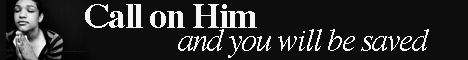Note:  Do not rely on this information. It is very old.

# Numbers

Numbers may be divided into two classes. If a number contains no factor greater than unity it is said to be prime, while one which is divisible by other numbers is said to be composite. Two numbers which have no common factor are said to be prime to each other. .From these definitions arise a number of elementary theorems, among which are the following: - (1) If a prime number, a, divide a product, bed, it must divide at least one of the factors, b, c, d; (2) if a number be prime to two other numbers, it must also be prime to their product; (3) if two numbers be prime to each other, their squares, cubes, etc., are also prime to each other; (4) a number cannot be decomposed into prime factors in more ways than one; (5) the number of primes is unlimited; (6) no formula can be found expressing prime numbers only. Numbers can be expressed by many different algebraic formulae which are useful for the determination of the properties of, and theorems relating to, numbers. Thus we can quite easily find the sum of any series of consecutive numbers starting from unity, or the sum of their squares or cubes, and still more simple is it to find the number of divisors which any number can have. The product of any two consecutive numbers is divisible by 2, the product of any three consecutive numbers is divisible by G, that of any four by 8, and so on. The theory of numbers is, however, very extensive, and includes the theory of equations, so that it forms an important branch of algebra. Numbers have been studied from very early times, while Fermat, Euler, Legendre, Lagrange, Gauss, Cauchy, etc., have greatly extended the science. Many theorems are known by the names of several of these illustrious mathematicians.